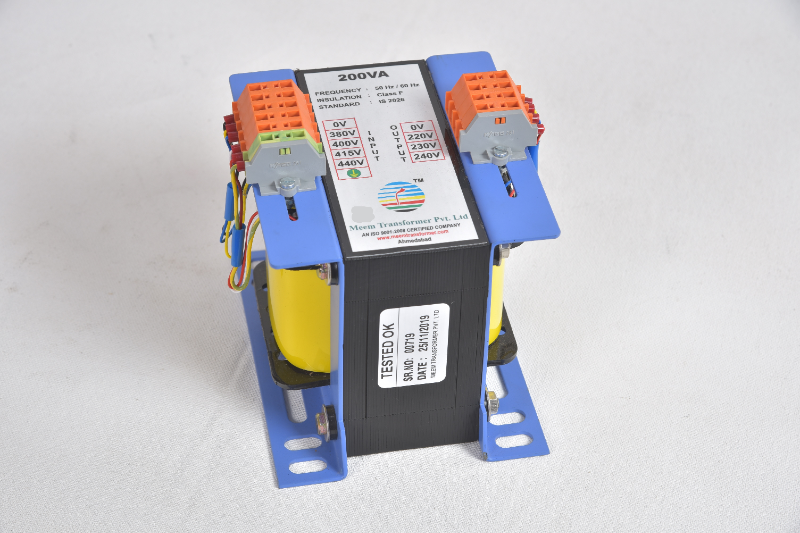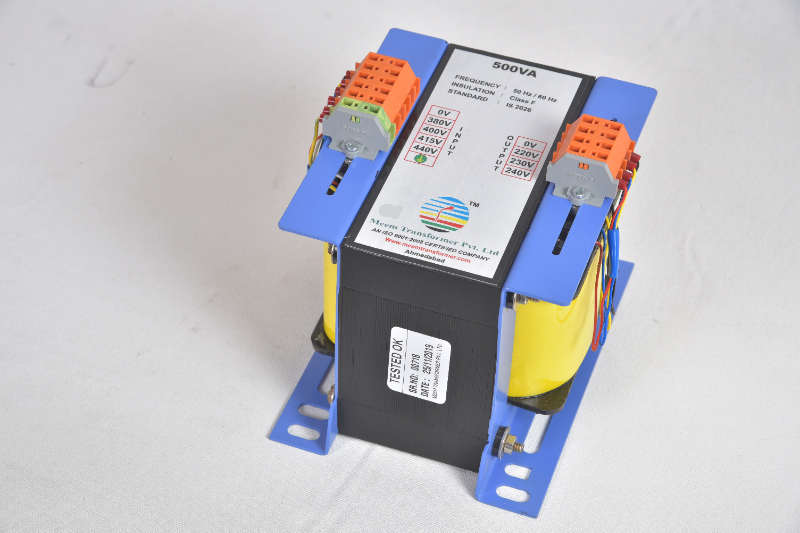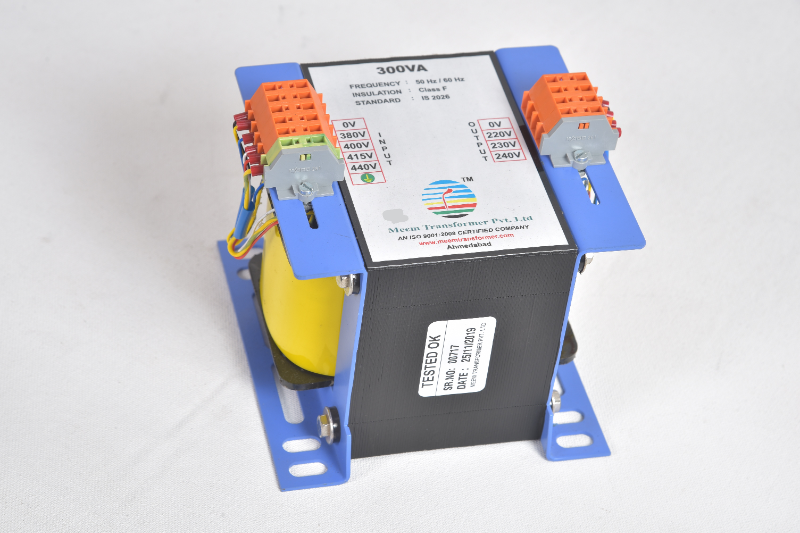Electric Control Transformer of 200 VA
₹ 1,534.00
Only 1 unit leftAhmedabad
Electric Control Transformer of 200 VA Transformer VA 200 VA Input Voltage : 0V-380V-400V-415V-440Volt-Earthing Output Voltage : 0V-220V-230V-240Volt Rate of Transfomer of 200 VA is = Rs. 1,300/-(Basic) + 18% GST Rate of Transfomer of 200 VA is = ...Electric Control Transformer of 500 VA
₹ 2,596.00
Only 1 unit leftAhmedabad
Electric Control Transformer of 500 VA Transformer VA 500 VA Input Voltage 0V-380V-400V-415V-440Volt-Earthing Output Voltage 0V-220V-230V-240Volt Rate of Transfomer of 500 VA is = Rs. 2,200/-(Basic) + 18% GST Rate of Transfomer of 500 VA is = Rs. 2...Electric Control Transformer of 300 VA
₹ 2,006.00
Only 1 unit leftAhmedabad
Electric Control Transformer of 300 VA Transformer of 300 VA Transformer VA 300 VA Input Voltage 0V-380V-400V-415V-440Volt-Earthing Output Voltage 0V-220V-230V-240Volt Rate of Transfomer of 300 VA is = Rs. 1,700/-(Basic) + 18% GST Rate of Transfome...Sounds Good ?
Dialog Title